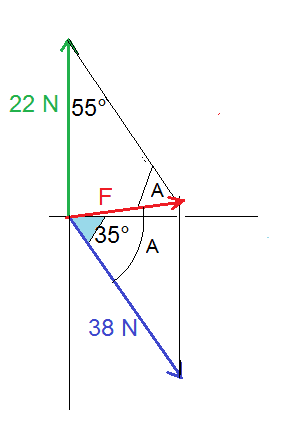# Finding Resultant Force Using Cosine and Sine Law

## Homework Statement

"Calculate the net force with the resultant angle acting on each object indicated in the diagram."

The line going off to the southeast is supposed to be straight. My computer art skills aren't great.

| 22 N
|
|_ _ _ _ _
\ ) 35 degrees
\
\ 38 N​

2. Homework Equations

Cosine and sine law

## The Attempt at a Solution

So the way the textbook teaches this is to redraw the diagram into a contained angle so that's what I did. I took the line that was 38 Newtons and shifted it so it still was in the exact same direction but now makes a contained angle with the 22 N force. I reckon that contained angle makes 55 degrees-- 180 - 35 - 90 = 55. I apologize for the word picture and not an actual picture. I don't know of a way to upload a picture of my work on paper on the forum.

From there I used cosine law (cosine and sine law is the method taught by my textbook to solve problems like this.)

## c^2 = a^2 + b^2 - 2ab Cos C
\\ = (38)^2 + (22)^2 - 2(38)(22) Cos C
\\ = \sqrt 968.98
\\ = 31.128##

Net force is 31 N

And sine law for the angle:

## {\frac {\sin A} a} = {\frac {\sin C} c}##

## {\frac {\sin A} {22}} = {\frac {\sin 55} {31}}##

Sin A = 0.581333708850252

The inverse = 35.54 or 36 degrees.

Now my textbook suggests that I need to subtract the original 35 degrees from this.

This makes the final answer Fnet = 31 N [ E 1 degree S]

Am I correct in any of this?

ehild
Homework Helper

## Homework Statement

"Calculate the net force with the resultant angle acting on each object indicated in the diagram."

The line going off to the southeast is supposed to be straight. My computer art skills aren't great.

| 22 N
|
|_ _ _ _ _
\ ) 35 degrees
\
\ 38 N​

2. Homework Equations

Cosine and sine law

## The Attempt at a Solution

So the way the textbook teaches this is to redraw the diagram into a contained angle so that's what I did. I took the line that was 38 Newtons and shifted it so it still was in the exact same direction but now makes a contained angle with the 22 N force. I reckon that contained angle makes 55 degrees-- 180 - 35 - 90 = 55. I apologize for the word picture and not an actual picture. I don't know of a way to upload a picture of my work on paper on the forum.

From there I used cosine law (cosine and sine law is the method taught by my textbook to solve problems like this.)

## c^2 = a^2 + b^2 - 2ab Cos C
\\ = (38)^2 + (22)^2 - 2(38)(22) Cos C
\\ = \sqrt 968.98
\\ = 31.128##

Net force is 31 N

And sine law for the angle:

## {\frac {\sin A} a} = {\frac {\sin C} c}##

## {\frac {\sin A} {22}} = {\frac {\sin 55} {31}}##

Sin A = 0.581333708850252

The inverse = 35.54 or 36 degrees.

Now my textbook suggests that I need to subtract the original 35 degrees from this.

This makes the final answer Fnet = 31 N [ E 1 degree S]

Am I correct in any of this?
You are correct in principle.
This is the figure showing the forces (blue and green) and the net force F (red).
Do not round off during the calculations. Rounding comes at the end when you give the result.You can draw with any drawing program you have (I use Paint of Windows). Then you can copy and paste into your post.

Thank you.